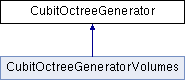cgma
CubitOctreeGenerator Class Reference

`#include <CubitOctreeGenerator.hpp>`

Inheritance diagram for CubitOctreeGenerator:List of all members.

## Public Member Functions

CubitOctreeGenerator ()
virtual ~CubitOctreeGenerator ()
CubitOctreeget_octree_lattice (void)
double size_at_point_in_octree (const CubitVector &point, int type)
double size_at_a_point (const CubitVector &point)
void color_lattice_cell (void)
virtual void get_bounding_box (CubitVector &min, CubitVector &max)=0
virtual CubitBoolean generate_lattice (void)=0
CubitPointContainment point_containment (CubitVector tmp_vec, double tolerance)

## Protected Attributes

CubitOctreecubitOctree

## Detailed Description

Definition at line 23 of file CubitOctreeGenerator.hpp.

## Constructor & Destructor Documentation

 CubitOctreeGenerator::CubitOctreeGenerator ( )

Definition at line 5 of file CubitOctreeGenerator.cpp.

```                                           {
}
```
 CubitOctreeGenerator::~CubitOctreeGenerator ( ) ` [virtual]`

Definition at line 8 of file CubitOctreeGenerator.cpp.

```                                           {
delete cubitOctree;
}
```

## Member Function Documentation

 void CubitOctreeGenerator::color_lattice_cell ( void )

Definition at line 12 of file CubitOctreeGenerator.cpp.

```                                                   {
cubitOctree->color_octreecell();
cubitOctree->set_status_octree_coloring(CUBIT_TRUE);
}
```
 virtual CubitBoolean CubitOctreeGenerator::generate_lattice ( void ) ` [pure virtual]`

Implemented in CubitOctreeGeneratorVolumes.

 virtual void CubitOctreeGenerator::get_bounding_box ( CubitVector & min, CubitVector & max ) ` [pure virtual]`

Implemented in CubitOctreeGeneratorVolumes.

 CubitOctree* CubitOctreeGenerator::get_octree_lattice ( void ) ` [inline]`

Definition at line 34 of file CubitOctreeGenerator.hpp.

```{ return cubitOctree;}
```
 CubitPointContainment CubitOctreeGenerator::point_containment ( CubitVector tmp_vec, double tolerance )

Definition at line 31 of file CubitOctreeGenerator.cpp.

```{
return cubitOctree->point_containment( tmp_vec, tolerance );
}
```
 double CubitOctreeGenerator::size_at_a_point ( const CubitVector & point )

Definition at line 17 of file CubitOctreeGenerator.cpp.

```{
double size = 0;

size = size_at_point_in_octree( point, MESH_SIZE );

return size;
}
```
 double CubitOctreeGenerator::size_at_point_in_octree ( const CubitVector & point, int type )

Definition at line 26 of file CubitOctreeGenerator.cpp.

```                                                                                         {
return cubitOctree->size_at_a_point( point, type );
}
```

## Member Data Documentation

 CubitOctree* CubitOctreeGenerator::cubitOctree` [protected]`

Definition at line 52 of file CubitOctreeGenerator.hpp.

The documentation for this class was generated from the following files: